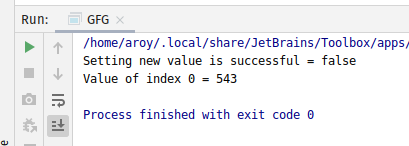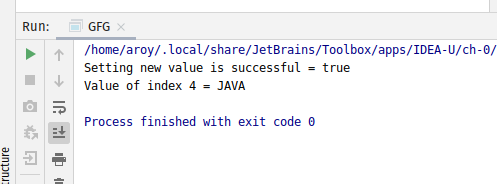# AtomicReferenceArray weakCompareAndSetAcquire() method in Java with Examples

• Last Updated : 03 Jan, 2020

The weakCompareAndSetAcquire() method of a AtomicReferenceArray class is used to atomically sets the value of the element at index i to newValue to newValue for AtomicReferenceArray if the current value is equal to expectedValue passed as parameter. This method updates the value at index i with memory ordering effects compatible with memory_order_acquire ordering. This method returns true if set a new value to AtomicRefrence is successful.

Syntax:

```public final boolean
weakCompareAndSetAcquire(
int i, E expectedValue, E newValue)
```

Parameters: This method accepts:

• i which is an index of AtomicReferenceArray to perform the operation,
• expectedValue which is the expected value and
• newValue which is the new value to set.

Return value: This method returns true if set a new value to AtomicRefrence is successful.

Below programs illustrate the weakCompareAndSetAcquire() method:
Program 1:

 `// Java program to demonstrate``// weakCompareAndSetAcquire() method`` ` `import` `java.util.concurrent.atomic.*;`` ` `public` `class` `GFG {`` ` `    ``public` `static` `void` `main(String[] args)``    ``{`` ` `        ``// create an atomic reference object.``        ``AtomicReferenceArray ref``            ``= ``new` `AtomicReferenceArray(``5``);`` ` `        ``// set some value``        ``ref.set(``0``, ``543``);``        ``ref.set(``1``, ``123``);``        ``ref.set(``2``, ``5443``);`` ` `        ``// apply weakCompareAndSetAcquire()``        ``boolean` `result``            ``= ref.weakCompareAndSetAcquire(``                ``0``,``                ``5443``,``                ``234``);`` ` `        ``// print value``        ``System.out.println(``            ``"Setting new value"``            ``+ ``" is successful = "``            ``+ result);`` ` `        ``System.out.println(``"Value of index 0 = "``                           ``+ ref.get(``0``));``    ``}``}`

Output:Program 2:

 `// Java program to demonstrate``// weakCompareAndSetAcquire() method`` ` `public` `class` `GFG {`` ` `    ``public` `static` `void` `main(String[] args)``    ``{`` ` `        ``// create an atomic reference object.c``        ``AtomicReferenceArray ref``            ``= ``new` `AtomicReferenceArray(``5``);`` ` `        ``// set some value``        ``ref.set(``0``, ``"C"``);``        ``ref.set(``1``, ``"PYTHON"``);``        ``ref.set(``2``, ``"TS"``);``        ``ref.set(``3``, ``"C++"``);``        ``ref.set(``4``, ``"C"``);`` ` `        ``// apply weakCompareAndSetAcquire()``        ``boolean` `result``            ``= ref.weakCompareAndSetAcquire(``                ``4``,``                ``"C"``,``                ``"JAVA"``);`` ` `        ``// print value``        ``System.out.println(``            ``"Setting new value"``            ``+ ``" is successful = "``            ``+ result);`` ` `        ``System.out.println(``            ``"Value of index 4 = "``            ``+ ref.get(``4``));``    ``}``}`

Output:My Personal Notes arrow_drop_up